From: Christopher I do not understand how to write the equation of a line with a slope of 3/8 and passing through (-4,6) in Ax+By=C. Hi Christopher. There are several forms that express a line and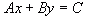is just one of them. Remember that A, B and C are all just numbers you need to figure out. If, as in this question, you are given the co-ordinates of a point that is on the line (-4, 6) and the slope, there is a better formula for a line that probably looks familiar to you: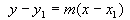In this form,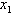and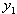are the co-ordinates that you know and m is the slope that you know. Let's plug your numbers in for these symbols: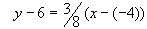Now you can simplify and re-arrange this into the formusing standard rules of algebraic equations (whatever you do to one side, you must do to the other). I'm pretty sure you can take it from here. Hope this helps! Stephen La Rocque.